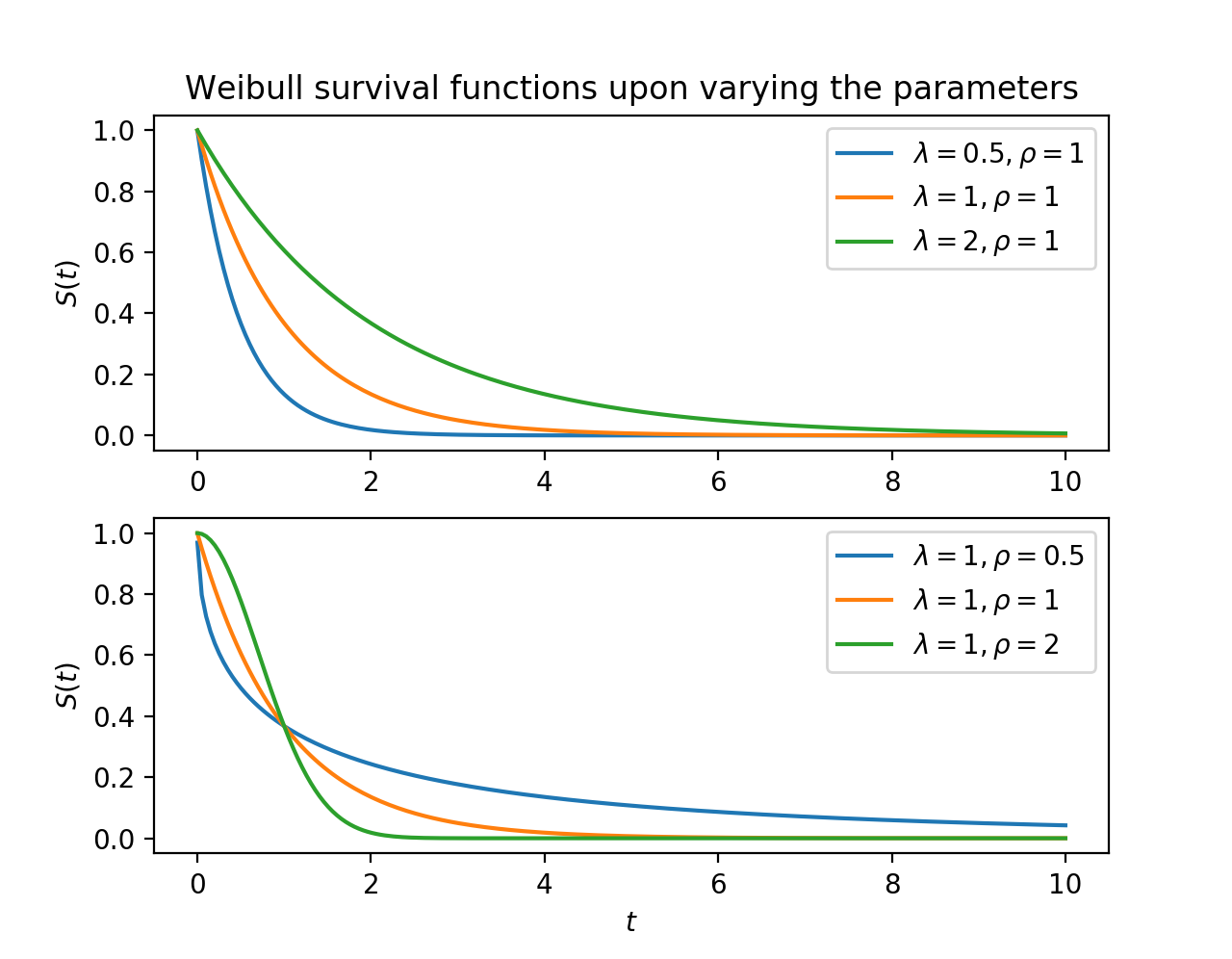# WeibullFitter¶

class lifelines.fitters.weibull_fitter.WeibullFitter(*args, **kwargs)

This class implements a Weibull model for univariate data. The model has parameterized form:

$S(t) = \exp\left(-\left(\frac{t}{\lambda}\right)^\rho\right), \lambda > 0, \rho > 0,$

The $$\lambda$$ (scale) parameter has an applicable interpretation: it represents the time when 63.2% of the population has died. The $$\rho$$ (shape) parameter controls if the cumulative hazard (see below) is convex or concave, representing accelerating or decelerating hazards.The cumulative hazard rate is

$H(t) = \left(\frac{t}{\lambda}\right)^\rho,$

and the hazard rate is:

$h(t) = \frac{\rho}{\lambda}\left(\frac{t}{\lambda}\right)^{\rho-1}$

After calling the .fit method, you have access to properties like: cumulative_hazard_, survival_function_, lambda_ and rho_. A summary of the fit is available with the method print_summary().

Parameters:

alpha (float, optional (default=0.05)) – the level in the confidence intervals.

Examples

from lifelines import WeibullFitter
wbf = WeibullFitter()
wbf.fit(waltons['T'], waltons['E'])
wbf.plot()
print(wbf.lambda_)

cumulative_hazard_

The estimated cumulative hazard (with custom timeline if provided)

Type:

DataFrame

hazard_

The estimated hazard (with custom timeline if provided)

Type:

DataFrame

survival_function_

The estimated survival function (with custom timeline if provided)

Type:

DataFrame

cumulative_density_

The estimated cumulative density function (with custom timeline if provided)

Type:

DataFrame

density_

The estimated density function (PDF) (with custom timeline if provided)

Type:

DataFrame

variance_matrix_

The variance matrix of the coefficients

Type:

DataFrame

median_survival_time_

The median time to event

Type:

float

lambda_

The fitted parameter in the model

Type:

float

rho_

The fitted parameter in the model

Type:

float

durations

The durations provided

Type:

array

event_observed

The event_observed variable provided

Type:

array

timeline

The time line to use for plotting and indexing

Type:

array

entry

The entry array provided, or None

Type:

array or None

Notes

Looking for a 3-parameter Weibull model? See notes here.

lambda_: float
percentile(p) float

Return the unique time point, t, such that S(t) = p.

Parameters:

p (float)

rho_: float